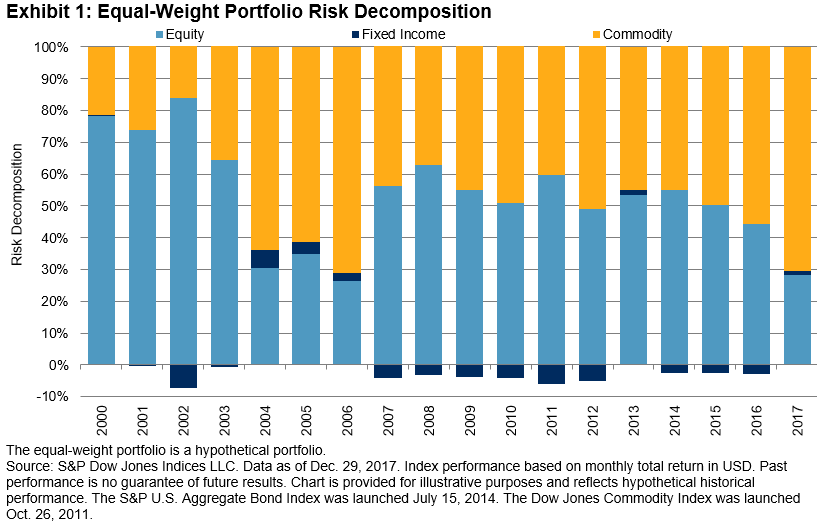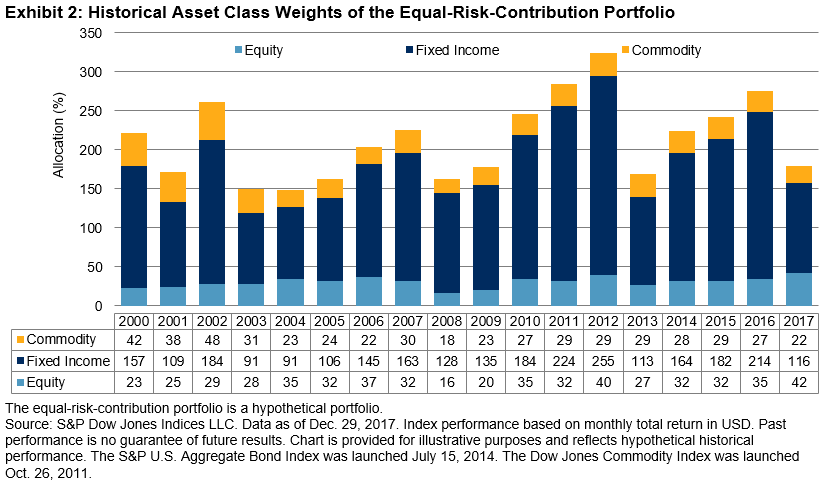# Equal-Weighting Versus Equal-Risk-Weighting Strategies

In a prior post, we reviewed the asset class risk contributions of a two-asset portfolio with varying weights. For an equal-weighted portfolio consisting of equities and bonds, we observed that nearly all contribution to total portfolio risk came from equities. To achieve equal risk contribution, the nominal weights in the portfolio would need to be closer 20% equities and 80% bonds. In this post, we extend the analysis by including the commodities asset class, and we observe how risk contributions change over time.

Commodities have relatively low correlations to traditional asset classes such as equities and bonds, thereby potentially increasing diversification when added to a multi-asset portfolio. Moreover, commodities generally perform well in periods of high growth and rising inflation.

Like equities, commodities have historically had relatively high return volatility. Hence, when combined in a three-asset portfolio with bonds, we anticipate that equities and commodities would contribute most to total portfolio volatility. For a portfolio that is equally weighted across the three asset classes, Exhibit 1 shows the risk contributions of each asset class on an annual basis, starting in 2000.For 2017, commodities contributed most to portfolio volatility, at 71%, significantly higher than its one-third weight allocation. Next, equities contributed second most, at 28%, while fixed income contributed just 1%. For the whole period, we observe that equities and commodities were the dominant contributors to total portfolio risk. On average, equities contributed 53% and commodities 48%—therefore, bonds negatively contributed (-1%) to the total risk. However, contributions varied from year to year; equities contributed as much as 84% to portfolio volatility in 2002, fixed income contributed 6% in 2004, and commodities contributed 71% in 2006 and 2017.

In order for the portfolio to be equal-risk weighted instead of equal weighted, the weight assigned to bonds would need to be markedly higher than the riskier asset classes. In fact, it is often necessary to incorporate leverage into the portfolio, where nominal weights of asset classes would sum to be more than 100%.

Next, we construct a basic equal-risk-contribution portfolio. The portfolio rebalances on an annual basis, with a target volatility level set to be equal to the equal-weighted portfolio volatility from the prior year.Exhibit 2 shows why leverage is needed for the portfolio, as the weight of the fixed income asset class often hovers above 100%. As the individual volatilities and cross-correlations of the asset classes vary, the nominal weights of the portfolio ranged from 149% to 324% over the entire period.

In a following post, we will review the performance differences between an equal-weighted three-asset portfolio and an equal-risk-weighted one.

   From Dec. 31, 1999 to Dec. 29, 2017, the annualized volatility of monthly returns were 14.5% for equities (S&P 500®), 16.2% for commodities (Dow Jones Commodity Index), and 3.6% for bonds (S&P U.S. Aggregate Bond Index).

   To construct the equal-risk-contribution portfolio, at the beginning of each calendar year, we used the past one year of daily returns to compute the marginal contribution to risk for each asset class. We employed an optimizer to determine the final set of weights such that each asset class contributed approximately one-third of the total portfolio volatility, subject to several constraints. We set the target portfolio volatility to be equal to the realized portfolio volatility of the equal-weight portfolio from the prior year, subject to a maximum of 10%. The portfolio is constrained to be long only (no negative weights or shorting). Lastly, using the three-month U.S. Treasury Bill as the borrow cost, leverage was allowed for fixed income. Hence, the total nominal weight of the portfolio exceeded 100%.

The posts on this blog are opinions, not advice. Please read our disclaimers.